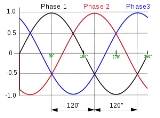xPolyphase systemOverview

A polyphase system is a means of distributing alternating current
Alternating current
In alternating current the movement of electric charge periodically reverses direction. In direct current , the flow of electric charge is only in one direction....

electrical power
Electric power
Electric power is the rate at which electric energy is transferred by an electric circuit. The SI unit of power is the watt.-Circuits:Electric power, like mechanical power, is represented by the letter P in electrical equations...

. Polyphase systems have three or more energized electrical conductors carrying alternating currents with a definite time offset
Phase (waves)
Phase in waves is the fraction of a wave cycle which has elapsed relative to an arbitrary point.-Formula:The phase of an oscillation or wave refers to a sinusoidal function such as the following:...

between the voltage waves in each conductor. Polyphase systems are particularly useful for transmitting power to electric motors. The most common example is the three-phase power system used for industrial applications and for power transmission.